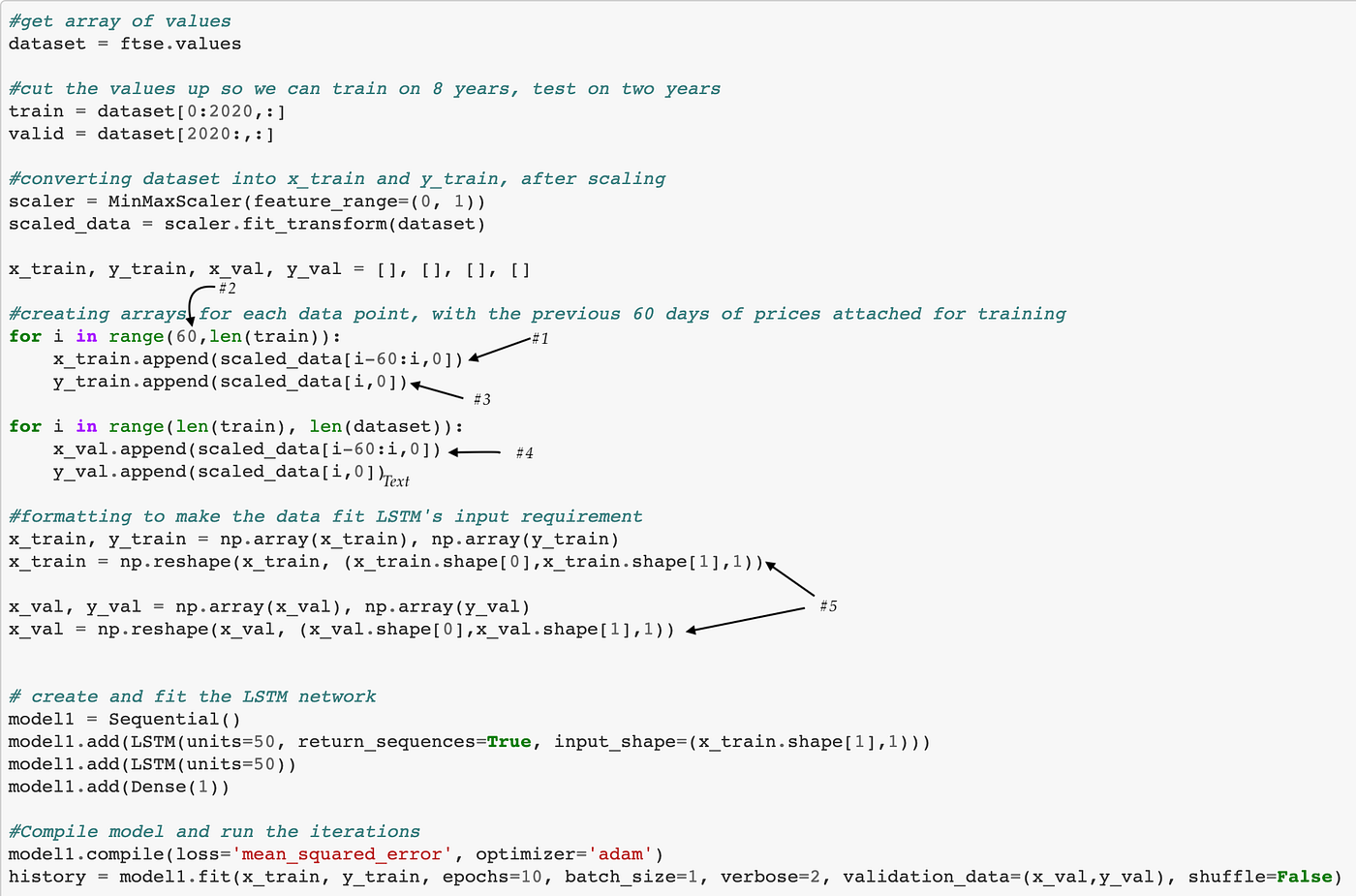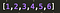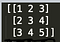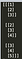Preparing your data for an LSTM model

If you’ve chosen an LSTM model to perform your time series modelling, then it’ll be a little bit of work to get your data prepared for training the model. Here’s how.

Firstly, remember that we use LSTM models because of their ability to ‘see’ patterns far back in time series data. The vanishing-gradient problem in other forms of time-series modelling results in models being less able to consider long-term patterns. LSTM models account for this.

Therefore, we expect our LSTM model will want to have the past data points readily available, and so it does. When we give the model a data point to learn from, it will want to have a copy of the past data points to learn from too.

The format that Keras requires is an array with three dimensions:

[‘Sample’, ‘Value at Timestep’, ‘Features’]

‘Features’: the number of features that our dataset has. Usually, this number is just one, like the price of gold, or the traffic to a website.

‘Value at Timestep’: the value of our feature at a particular timestep. Just one number, like the value of Apple Stock on December 20th 2019.

‘Sample’: a sample of the past data points. For example, the price of Apple stock in the week previous to December 20th 2019.

Run through all of your data points, where each data point has a copy of the data points that came before it.

Let’s look at an example from a model I made to predict the FTSE price:

After splitting up the dataset of past FTSE values into training and validation sets, and scaling, we’re ready to start.

Note, the number 2020 is a coincidence; it is just 80% of the way into our dataset, leaving the last 20% as a validation set.Example of code for an LSTM Model — follow along with the numbers below for more details

1— We create an array, x_train, where every data point is a list. Each list is the previous 60 days prices for all the dates in our dataset.

2— Note, we have to start on day 61 to work, so we ‘lose’ the first 60 days of data.

3 — We create a new array, y_train, the FTSE's current price over our dates.

So, x-train contains the “historical” and y_train includes the “current” prices.

4 — We also create x_val and y_val, which are validation sets, on our dataset's later dates.

5 — We take our datasets x_train and x_val, and reshape them to be 3D from 2D. Note, this is only reformatting the data; it isn’t adding any new data.

6— There is no need to reshape the y_train or y_val arrays, as the model does not require the y values to be in this 3D shape.

And voila! You can choose your loss functions and optimiser as you see fit, but in this format, your model will run, train and produce the results you need.Let's assume that list was our FTSE prices over time.This would be x_train before we reshape it, so it's 2D (3,3)This is what x_train looks like after we reshape it to 3D (3,3,1)

If the different shapes of the training sets prove confusing, here is a more straightforward example to follow.

Our price list is an array.

Then, we make a new array, that contains the price ‘histories.’

Finally, we a new array, that contains the price histories, where each individual price is an array, so each data point is an array of an array.